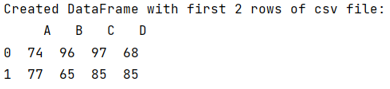# How to Read First N Rows from DataFrame in Pandas?

Given a Pandas DataFrame, we have to read first N rows from it.
Submitted by Pranit Sharma, on June 20, 2022

Rows in pandas are the different cell (column) values that are aligned horizontally and also provide uniformity. Each row can have the same or different value. Rows are generally marked with the index number but in pandas, we can also assign index names according to the needs. In pandas, we can create, read, update and delete a column or row value.

To read the first N rows of a DataFrame, we will use a parameter called nrows at the time of reading s CSV file by using pandas.read_csv() method.

To work with pandas, we need to import pandas package first, below is the syntax:

```import pandas as pd
```

Let us understand with the help of an example,

## Python code to Read First N Rows from DataFrame in Pandas

```# Importing pandas package
import pandas as pd

# Creating a Dataframe with first 2 rows
df = pd.DataFrame(data)

# Display Dataframe
print("Created DataFrame with first 2 rows of csv file:\n",df)
```

Output:Preparation

What's New

Top Interview Coding Problems/Challenges!